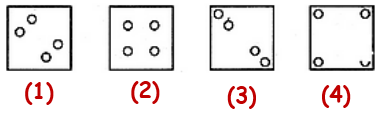# Paper Cutting Interview Questions and Answers Part 1

## Above video will provide solutions for below questions

1. Each of the following questions consists of a set of three figures X, Y and Z showing a sequence of folding of a piece of paper. Figure (Z) shows the manner in which the folded paper has been cut. These three figures are followed by four answer figures from which you have to choose a figure which would most closely resemble the unfolded form of figure (Z).

2. Choose a figure which would most closely resemble the unfolded form of Figure (Z).1. 1
2. 2
3. 3
4. 4